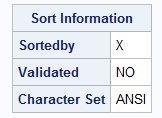## create a VALIDATESORT data set option to validate the sort order as the data set is written

``````data T(sortedby=X);
X=1; output;
X=2; output;
run;``````

will not set the validated flag in the data set's metadata as seen here:One must recreate the table using for example proc sort to set this flag. This is hugely wasteful.

The following option would ask SAS to check the sort order as the data is written, in order to avoid this waste:

``````
data T(sortedby=X validatesort);
X=1; output;
X=2; output;
run;``````Opal | Level 21

Good idea. Would also be useful when importing text file data that is supposed to be sorted.

But then, you also need some way to take action if the validation fails. An automatic macro variable wouldn't  be a good idea since many datasets may be produced by a single data step. Maybe VALIDATESORT=WARNING, VALIDATESORT=ERROR.Tourmaline | Level 20
Even a note reading that the validation failed. The error will come at the next step when the data isn't as expected.Tourmaline | Level 20

Actually, thinking about it, the VALIDATESORT should not be needed.

SAS should be clever enough to check output order when needed:

- when the input data set(s) is(are) sorted (by the same key)

- when a BY statement is used

- when option SORTEDBY is used

No code change needed.

Sorted tables is THE major performance differentiator between SAS tables and database tables. And SAS doesn't leverage it.Tourmaline | Level 20

The same should happen here. Why aren't the sorted flags set when this code is run:

``````proc summary data=SASHELP.CLASS ; * sorted by _TYPE_ SEX;
class SEX;
var WEIGHT;
output out=T;
proc contents;
run;

proc summary data=SASHELP.CLASS nway;  * sorted by SEX;
class SEX;
var WEIGHT;
output out=T;
proc contents;
run;

proc freq data=SASHELP.CLASS order=internal; * sorted by SEX;
table SEX / out=T;
proc contents;
run;

data _NULL_;
WEIGHT=.;
dcl hash H(dataset:'SASHELP.CLASS', ordered:'A');
H.definekey('WEIGHT');
H.definedone();
H.output(dataset:'T');    * sorted by WEIGHT;
run;``````